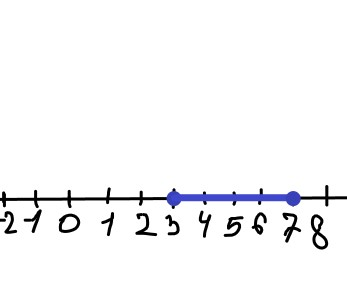# Express the graph shown in color using interval notation. Also express it as an inequality involving x. Use interval notation to describe the graph. What is the resulting interval?Raegan Bray 2022-08-01 Answered
Express the graph shown in color using interval notation. Also express it as an inequality involving x.Use interval notation to describe the graph. What is the resulting interval?
You can still ask an expert for help

• Questions are typically answered in as fast as 30 minutes

Solve your problem for the price of one coffee

• Math expert for every subject
• Pay only if we can solve itJulianna Bell
Step 1
The graph starts from 3 (including)
Step 2
$\therefore 3\le x\le 7$
$x\in \left[3,7\right]$